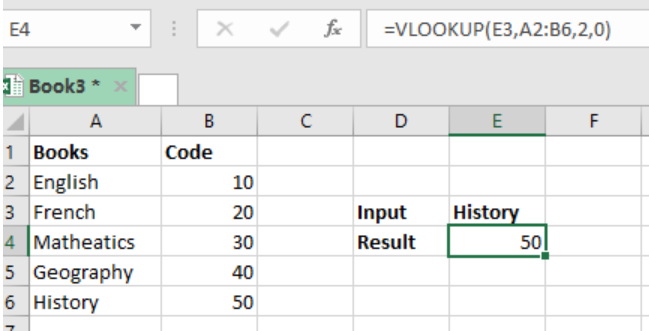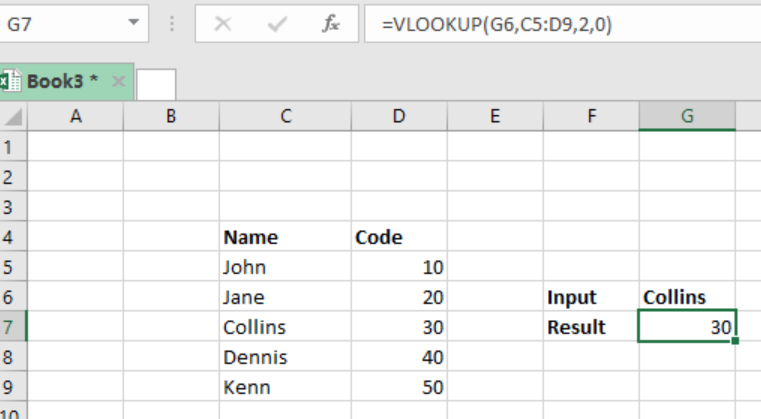Get instant live expert help with Excel or Google Sheets“My Excelchat expert helped me in less than 20 minutes, saving me what would have been 5 hours of work!”

#### Post your problem and you’ll get Expert help in seconds.

Your message must be at least 40 characters
Our professional Expert are available now. Your privacy is guaranteed.

# How to Use VLOOKUP to Map Text to Numbers

Translating text inputs into arbitrary numeric values might look easy if we are dealing with a small set of values, but what if the list of values is so big? Learning how to map text to numbers in excel is a great step towards making your work easy and simple. This article provides a clear guide on how you can use the VLOOKUP function to map text to numbers in excel.Figure 1: How to map text to numbers

## General syntax of the formula

`=VLOOKUP(text,lookup_table,2,0)`

## Understanding the formula

The use of the VLOOKUP function is the only sure way we can get the arbitrary numeric values from text strings. In the above table, we are trying to get the arbitrary numeric values of the books, which have been assigned unique codes.

## How the formula works

• The formula utilizes the VLOOKUP function to translate text to numbers.
• In our case above, the formula is using the value in cell E4 as the lookup value, A2:B6 as the range for the lookup table, 2 to indicate the 2nd column and zero to force exact match.
• After we have entered the formula in cell E4, we press enter to get the numeric value, which is the code for History.
• Note that this formula can also be used to map text to text as well as numbers to text.

### ExampleFigure 2: Example of how to map text to numbers in excel

In this example, we have names of individuals and their unique codes. We want to map the names to their respective codes. To do this, we proceed as follows:

Step 1: Fill the table with the data I.e. name and code

Step 2: Indicate where you want the result to be displayed

Step 3: Put the formula in the cell where you want to have the result, `=VLOOKUP(G6,C5:D9,2,0)`

Step 4: Press Enter to get the arbitrary numeric value of the text.

## Instant Connection to an Expert through our Excelchat Service

Most of the time, the problem you will need to solve will be more complex than a simple application of a formula or function. If you want to save hours of research and frustration, try our live Excelchat service! Our Excel Experts are available 24/7 to answer any Excel question you may have. We guarantee a connection within 30 seconds and a customized solution within 20 minutes.

### Did this post not answer your question? Get a solution from connecting with the expert.Another blog reader asked this question today on Excelchat:
Solution examplesI have a pivot table in which per order (on the rows) stands how much products they ordered per size (on the columns). I want to determine which combinations of quantities of sizes people order. And I want to count these combinations.
Solved by E. W. in 60 minsI have 500 numbers in column A with aproximatly 15digits. In column B I have 5 numbers with 6 digits Can a find a formula wich can transfer all numbers from A column wich start with some 6 digitsa from B column
Solved by V. L. in 32 minsI have a sheet with 3 columns. First column is a code for records in column B (has 563 records). What I need is a formula to show me if what is in column C (has 4400 records) is in column B and if it is to take the code for that record. Example: Column A(code corresponding to column B): 12, 14, 15, 19 Column B(names): asd, adf, ade, aqw Column C(names): akd, adf, ade, anb, wgs I need something that would say, if record in column C (for example adf) is matching record in column B (adf) return the code from column A (the code corresponding to that record in column B),if not return blank.
Solved by C. J. in 8 minsIf the data in A matches the data in C, then I need the data in E to be placed in B
Solved by X. W. in 40 minsI have a workbook with 2 sheets. First sheet has column A which is a device name, then columns B - infinity that have numeric "tags" for that device. Each device can have one or 20 "tags", tags are all numerical. Worksheet 2 has column A which is numeric value and B which is a human readable value of what the tag is. Question is, how do I replace all the tag numbers in worksheet 1 with the actual human readable values is sheet 2?
Solved by T. H. in 16 mins## Subscribe to Excelchat.coAnother blog reader asked this question today on Excelchat: